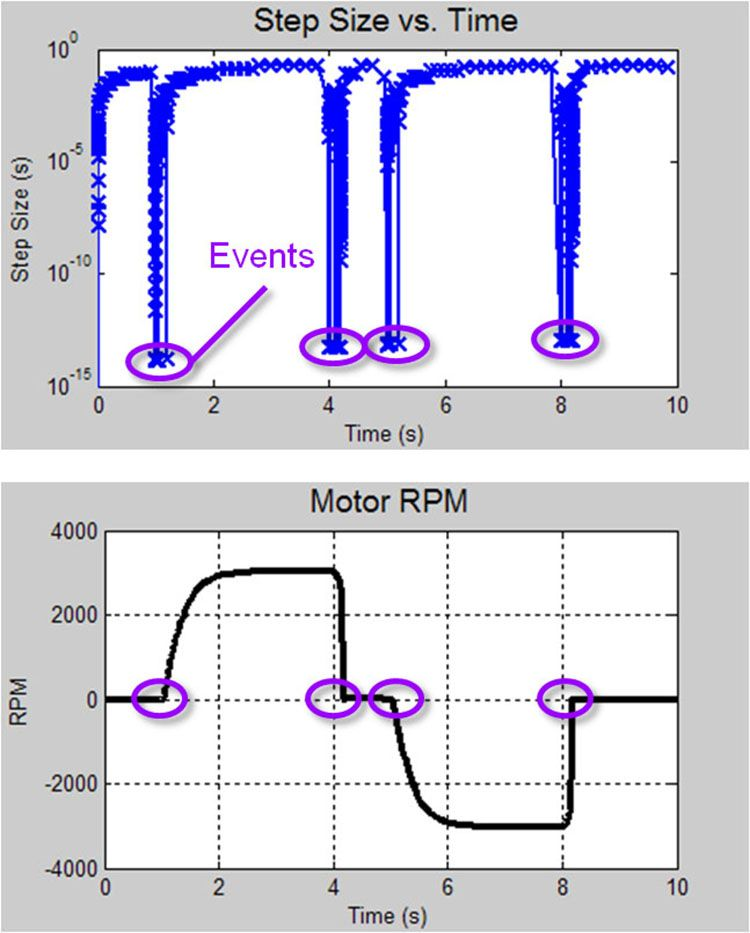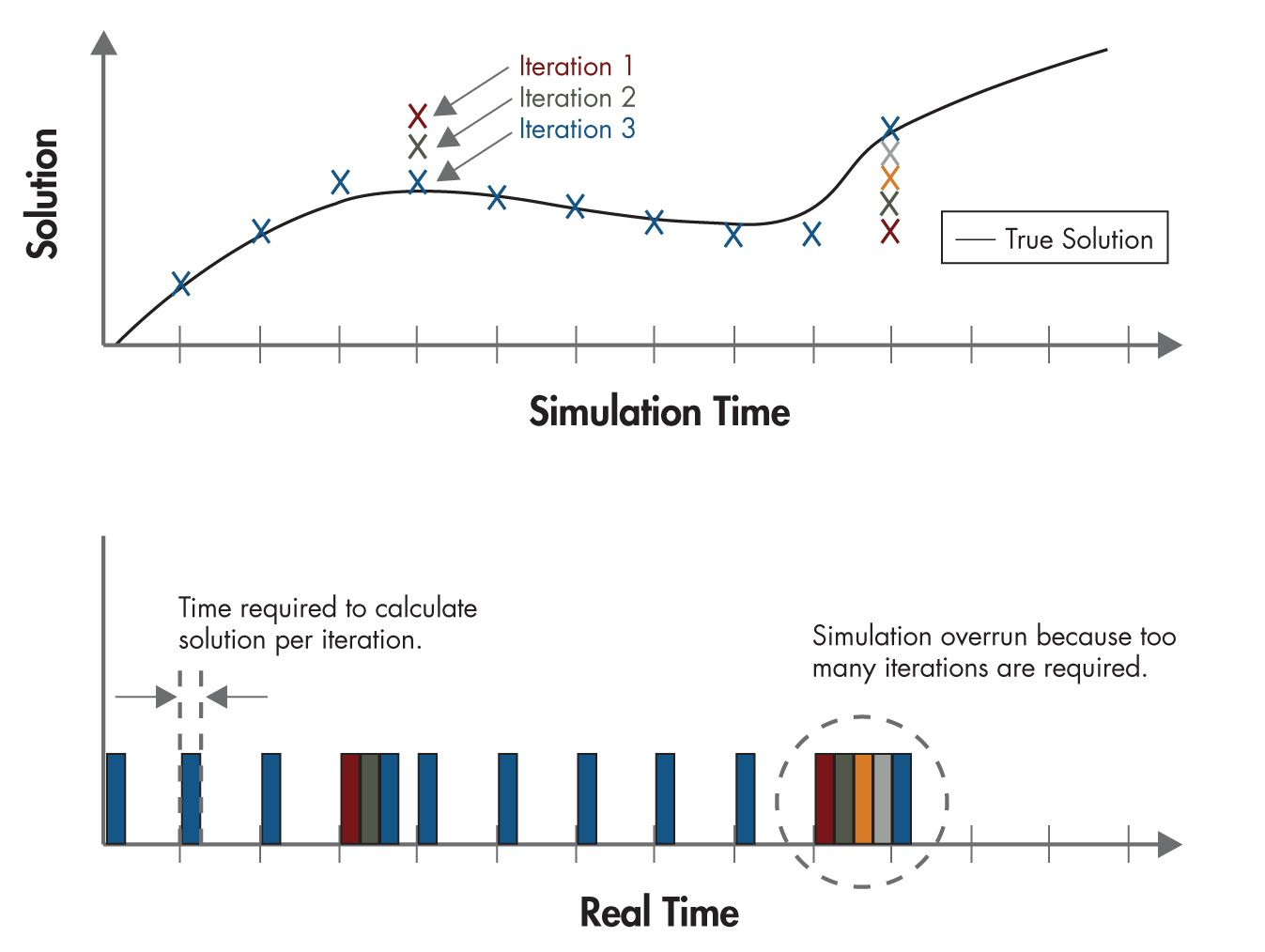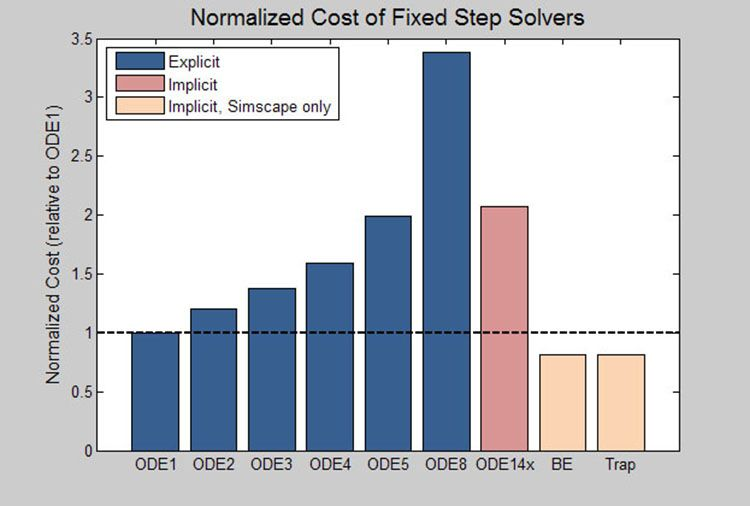Real-Time Simulation of Physical Systems Using Simscape

By Steve Miller, MathWorks and Jeff Wendlandt, MathWorks

Replacing a physical system like a vehicle, plane, or robot with a real-time simulation of a virtual system drastically reduces the cost of testing control software and hardware. In real-time simulation, the inputs and outputs in the virtual world of simulation are read or updated synchronously with the real world. When the simulation time reaches 5, 50, or 500 seconds, exactly the same amount of time has passed in the real world. Testing can take place 24 hours a day, 7 days a week, under conditions that would damage equipment or injure personnel, and it can begin well before physical prototypes are available.

Real-time simulation of physical systems requires finding a combination of model complexity, solver type, solver settings, and simulation hardware that permits execution in real time and delivers results sufficiently close to the results obtained from desktop simulation. Changing these items will often speed up the simulation but reduce the accuracy, or vice-versa. Simscape™ provides several capabilities that make it easier to configure your models for real-time simulation. This article shows how to configure a Simscape model of a pneumatic actuation system for real-time simulation (Figure 1). The steps described apply regardless of the real-time hardware used.

Moving from Desktop to Real-Time Simulation

Configuring the Simscape model for real-time simulation involves five steps:

1. Obtain reference results with a variable-step solver.
2. Examine the step sizes during simulation.
3. Choose a fixed-step solver and configure a fixed-cost simulation.
4. Find the right balance between accuracy and simulation speed.
5. Simulate the model on the real-time platform.

1. Obtaining Reference Results with a Variable-Step Solver

A variable-step solver shrinks the step size to accurately capture events and dynamics in the system. Since this reduces the amount of time that the real-time target has to calculate the results for that time step, a variable-step solver cannot be used for real-time simulation. Instead, an implicit or explicit fixed-step solver must be used.

To ensure that the results obtained with the fixed-step solver are accurate, we compare them with reference results obtained by simulating the system with a variable-step solver and tightening the error tolerances until the simulation results stop changing (Figure 2). The recommended variable-step solvers for Simscape models are ode15s and ode23t.

2. Examining the Step Sizes During Simulation

A variable-step solver varies the step size to stay within the error tolerances and to react to zero crossing events. If the solver abruptly reduces the step size to a small value, such as 1e-15s, this indicates that the solver is trying to accurately identify a zero crossing event such as a reverse in flow or closing of a switch. A fixed-step solver may have trouble capturing these events at a step size large enough to permit real-time simulation.

We use the following MATLAB® commands to generate a plot showing how the time step varies during the simulation:

semilogy
(tout(1:end-1),diff(tout),’-*’);
title
(‘Step Size vs. Simulation Time’,’F
ontSize’,14,’FontWeight’,’bold’);
xlabel
(‘Simulation Time(s)’,’FontSize’,12);
ylabel
(‘Step Size(s)’,’FontSize’,12);

The plot indicates that we should adjust the friction model (see Figure 1, Friction Load) to make the system model real-time capable (Figure 3).Figure 3. Top: Plot of step size during simulation. Bottom: Plot of motor speed. The zero crossing events coincide with the speed of the motor approaching or leaving 0 RPM, indicating that the friction model may need to be adjusted to make the system model real-time capable.

3. Choosing a Fixed-Step Solver and Configuring a Fixed-Cost Simulation

We must use a fixed-step solver that provides robust performance and delivers accurate results at a step size large enough to permit real-time simulation. We compare the simulation results generated by an implicit fixed-step solver and an explicit fixed-step solver for the same model at different step sizes (Figure 4). For our example model, the implicit solver provides more accurate results.Figure 4. Plot comparing simulation results when the model is simulated with a variable-step solver, an implicit fixed-step solver, and an explicit fixed-step solver. The explicit solver requires a smaller time step than an implicit solver to achieve a comparable level of accuracy.

For real-time simulation, overruns that occur when the execution time is longer than the sample time must be prevented (Figure 5). To do this, we run a fixed-cost simulation, limiting the number of iterations per time step.Figure 5. How an overrun occurs. Top: More iterations are sometimes required to obtain an accurate solution. Bottom: Simulation overrun occurs because iterations can't be completed within the time step, which is held constant for a fixed-step simulation.

As Figure 5 shows, iterations are often necessary for each Simscape physical network for both explicit and implicit solvers. The iterations in Simscape are limited by setting the checkbox “Use fixed-cost runtime consistency iterations” and entering the number of nonlinear iterations in the Solver Configuration block (Figure 6). For the best balance between accuracy and cost, we recommend initially setting the number of nonlinear iterations to two or three.

To indicate the relative cost for the available fixed-step solvers, we compare the normalized execution time for a nonlinear model containing a single Simscape physical network with each fixed-step solver (Figure 7). In our example, the two local Simscape solvers, Backward Euler and Trapezoidal Rule, require the least computational effort.BE = Backward Euler, Trap = Trapezoidal Rule Figure 7. Plot of the normalized cost of all fixed-step solvers that can be used on Simscape models. The results were obtained by simulating a nonlinear model containing a single Simscape physical network with each solver at the same step size and similar settings for the total number of solver iterations.

By using the local solver option in Simscape, we can use an implicit fixed-step solver on the stiff portions of the model and an explicit fixed-step solver on the remainder of the model (Figure 8). This minimizes the number of computations performed per time step, making it more likely that the model will run in real time.Figure 8. Diagram showing use of multiple solvers on a single model. Using local solvers lets you configure implicit solvers on the stiff portions of the model and explicit solvers on the remainder of the model, minimizing execution time while maintaining accuracy.

4. Balancing Accuracy and Simulation Speed

We can now run the simulation using the C code that will run on the actual processor. During each time step, the real-time system reads the inputs, calculates the simulation results for the next time step, and writes the outputs. If this task takes less time than the specified time step, the processor remains idle during the remainder of the step.

The challenge is to find settings that provide accurate results while permitting real-time simulation (Figure 9). In each case, there is a tradeoff of accuracy versus speed. Choosing a computationally intensive solver, increasing the number of nonlinear iterations, or reducing the step size increases accuracy and reduces idle time, raising the risk that the simulation will not run in real time. On the other hand, adjusting these settings in the opposite direction increases the amount of idle time but reduces accuracy.Figure 9. The tradeoff involving solver choice, number of nonlinear iterations, and step size. For any given model, these settings must be chosen to deliver maximum accuracy and robustness with enough idle time to provide sufficient safety margin.

By using the Simscape Backward Euler local solver and limiting the number of iterations to two, we obtain accurate simulation results for our Simscape model at an acceptable simulation speed.

5. Simulating the Model on the Real-Time Platform

Our model ran in real time on a 700 MHz processor and used only 62% of the available step size to perform the necessary calculations. The results obtained from the real-time simulation were identical to those obtained using the same solver settings during the desktop simulation. These results were also very close to the reference results obtained using a variable-step solver (Figure 10).Figure 10. Plot comparing reference results obtained with a variable-step solver and simulation results after configuring for real-time simulation.

Extending This Approach

The approach described in this article is not limited to one type of model. We applied this approach to more than 30 models spanning a range of applications and physical domains. These models contain hydraulic, electrical, mechanical, pneumatic, and thermal elements, and include applications such as hydromechanical servovalves, brushless DC motors, hydraulic pipelines with water hammer effects, and pneumatic actuation systems with stick-slip friction. All models were able to run in real time on an Intel® Core 2 Duo E6700 (2.66 GHz) that was running xPC Target™. The maximum percentage of a step spent in simulation execution was less than 18%, leaving a wide safety margin for processing I/O and other tasks. The average percentage spent in simulation execution was 3.9%, and the minimum was 6e-4%.

Published 2011 - 91881v00